# opencv6.3-imgproc图像处理模块之边缘检测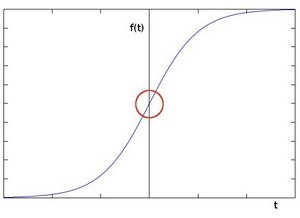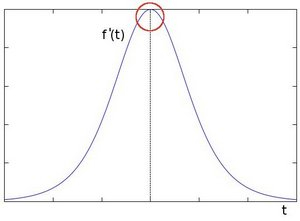1、sobel算子

Sobel 算子是一个离散微分算子 (discrete differentiation operator)。 它用来计算图像灰度函数的近似梯度。

Sobel 算子结合了高斯平滑和微分求导。

1. 在两个方向求导:

1. 水平变化: 将I与一个奇数大小的内核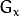进行卷积。比如，当内核大小为3时,的计算结果为: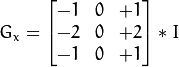b.垂直变化: 将:math:I与一个奇数大小的内核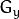进行卷积。比如，当内核大小为3时,的计算结果为: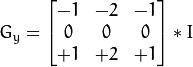2.在图像的每一点，结合以上两个结果求出近似 梯度: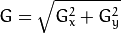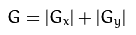Notes:当内核大小为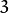时, 以上Sobel内核可能产生比较明显的误差(毕竟，Sobel算子只是求取了导数的近似值)。 为解决这一问题，OpenCV提供了 Scharr 函数，但该函数仅作用于大小为3的内核。该函数的运算与Sobel函数一样快，但结果却更加精确，其内核为: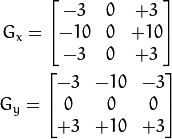int scale = 1;
int delta = 0;
int ddepth = CV_16S;
// 创建 grad_x 和 grad_y 矩阵
Mat abs_grad_x, abs_grad_y;
//示例代码中是先图像进行高斯平滑的 然后在转换成灰度图
//src_gray 为读取的图像转换成灰度图
  /// 求 X方向梯度
//Scharr( src_gray, grad_x, ddepth, 1, 0, scale, delta, BORDER_DEFAULT );
Sobel( src_gray, grad_x, ddepth, 1, 0, 3, scale, delta, BORDER_DEFAULT );

/// 求Y方向梯度
//Scharr( src_gray, grad_y, ddepth, 0, 1, scale, delta, BORDER_DEFAULT );
Sobel( src_gray, grad_y, ddepth, 0, 1, 3, scale, delta, BORDER_DEFAULT );

/// 合并梯度(近似)



– src.depth() = CV_8U, ddepth = -1/CV_16S/CV_32F/CV_64F
– src.depth() = CV_16U/CV_16S, ddepth = -1/CV_32F/CV_64F
– src.depth() = CV_32F, ddepth = -1/CV_32F/CV_64F
– src.depth() = CV_64F, ddepth = -1/CV_64F

ksize:默认情况下没有缩放；必须是正的，而且是奇数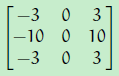sobel函数是通过对图像使用一个合适的核来卷积来进行求导的：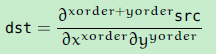sobel算子是结合了高斯平滑和微分的，所以结果或多或少对噪音具有不变性。最多的这个函数会使用（xorder = 1，yorder = 0，ksize=3）或者（xorder = 0，yorder = 1，ksize = 3）来计算第一次的x方向或者y方向的导数。

2、laplace算子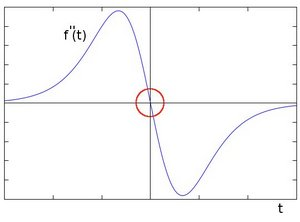Laplacian 算子 的定义: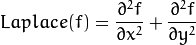OpenCV函数 Laplacian 实现了Laplacian算子。 实际上，由于 Laplacian使用了图像梯度，它内部调用了 Sobel 算子。

Mat src, src_gray, dst;
int kernel_size = 3;
int scale = 1;
int delta = 0;
int ddepth = CV_16S;//因为输入图像的深度是 CV_8U ，这里我们必须定义 ddepth = CV_16S 以避免外溢。

GaussianBlur( src, src, Size(3,3), 0, 0, BORDER_DEFAULT );
/// 转换为灰度图
cvtColor( src, src_gray, CV_RGB2GRAY );
/// 使用Laplace函数
Mat abs_dst;
Laplacian( src_gray, dst, ddepth, kernel_size, scale, delta, BORDER_DEFAULT );
convertScaleAbs( dst, abs_dst );
/// 显示结果
imshow( window_name, abs_dst );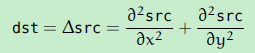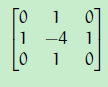ps：想要知道如何使用sobel来实现这个的，就需要看他的cpp实现代码了，暂时没去找。

3、canny边缘检测

Canny 边缘检测算法 是 John F. Canny 于 1986年开发出来的一个多级边缘检测算法，也被很多人认为是边缘检测的 最优算法, 最优边缘检测的三个主要评价标准是:

a、消除噪声。 使用高斯平滑滤波器卷积降噪。 下面显示了一个 size=5的高斯内核示例：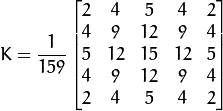b、计算梯度幅值和方向。 此处，按照Sobel滤波器的步骤:

i）运用一对卷积阵列 (分别作用于 x 和 y 方向):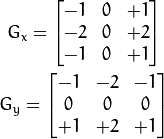ii）使用下列公式计算梯度幅值和方向: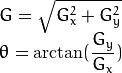梯度方向近似到四个可能角度之一(一般 0, 45, 90, 135)

c、非极大值 抑制。 这一步排除非边缘像素， 仅仅保留了一些细线条(候选边缘)。

d、滞后阈值: 最后一步，Canny 使用了滞后阈值，滞后阈值需要两个阈值(高阈值和低阈值):

i）如果某一像素位置的幅值超过  阈值, 该像素被保留为边缘像素。

ii）如果某一像素位置的幅值小于  阈值, 该像素被排除。

iii）如果某一像素位置的幅值在两个阈值之间,该像素仅仅在连接到一个高于  阈值的像素时被保留。

Canny 推荐的 : 阈值比在 2:1 到3:1之间。

e、想要了解更多细节，你可以参考任何你喜欢的计算机视觉书籍。

Mat dst, detected_edges;

int edgeThresh = 1;
int lowThreshold;
int const max_lowThreshold = 100;
int ratio = 3;
int kernel_size = 3;
/// 原图像转换为灰度图像
cvtColor( src, src_gray, CV_BGR2GRAY );

/// 使用 3x3内核降噪
blur( src_gray, detected_edges, Size(3,3) );
/// 运行Canny算子
Canny( detected_edges, detected_edges, lowThreshold, lowThreshold*ratio, kernel_size );
/// 创建与src同类型和大小的矩阵(dst)
dst.create( src.size(), src.type() );  /// 使用 Canny算子输出边缘作为掩码显示原图像  
dst = Scalar::all(0);  src.copyTo( dst, detected_edges);///copyTo 将 src 图像拷贝到 dst . 但是，仅仅拷贝掩码不为0的像素。既然Canny边缘检测的输出是镶嵌在黑色背景中的边缘像素，因此其结果 dst图像除了被检测的边缘像素，其余部分都为黑色  imshow( window_name, dst );

1、线检测

a、众所周知, 一条直线在图像二维空间可由两个变量表示. 例如:

i）在 笛卡尔坐标系: 可由参数: （m，b）斜率和截距表示.

ii）在 极坐标系: 可由参数: （r，theta）极径和极角表示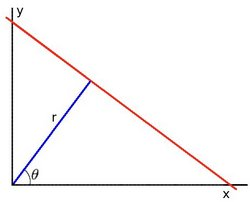对于霍夫变换, 我们将用 极坐标系 来表示直线. 因此, 直线的表达式可为: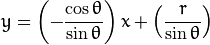化简得: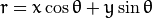b、一般来说对于点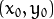, 我们可以将通过这个点的一族直线统一定义为: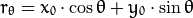这就意味着每一对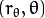代表一条通过点的直线

c、如果对于一个给定点我们在极坐标对极径极角平面绘出所有通过它的直线, 将得到一条正弦曲线. 例如, 对于给定点        x0=8 and y0=6 我们可以绘出下图 (在平面 theta-r):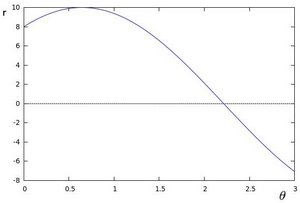只绘出满足下列条件的点 r >0 和 0<theta <2*pi；

d、我们可以对图像中所有的点进行上述操作. 如果两个不同点进行上述操作后得到的曲线在平面 theta-r 相交, 这就意味着它们通过    同一条直线. 例如, 接上面的例子我们继续对点:x1 = 9，y1 = 4和点x2 = 12，y2 = 13画图, 得到下图: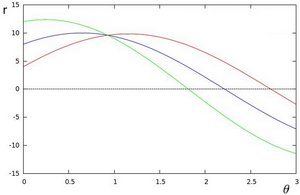这三条曲线在 （theta - r ）平面相交于点 （0.925，9.6）， 坐标表示的是参数对（theta ，r） 或者是说点（x0，y0），点      （x1，y1） 和点（x2，y2）  组成的平面内的的直线.

e、那么以上的材料要说明什么呢? 这意味着一般来说, 一条直线能够通过在平面 theta-r 寻找交于一点的曲线数量来 检测. 越多曲    线交于一点也就意味着这个交点表示的直线由更多的点组成. 一般来说我们可以通过设置直线上点的 阈值 来定义多少条曲线交于    一点我们才认为 检测 到了一条直线.

f、这就是霍夫线变换要做的. 它追踪图像中每个点对应曲线间的交点. 如果交于一点的曲线的数量超过了 阈值, 那么可以认为这个交    点所代表的参数对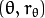在原图像中为一条直线.

OpenCV实现了以下两种霍夫线变换:

a、标准霍夫线变换：原理在上面的部分已经说明了. 它能给我们提供一组参数对的集合来表示检测到的直线；在OpenCV 中通过函数 HoughLines 来实现

b、统计概率霍夫线变换：这是执行起来效率更高的霍夫线变换. 它输出检测到的直线的端点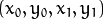在OpenCV 中它通过函数 HoughLinesP 来实现

 Mat dst, cdst;
Canny(src, dst, 50, 200, 3);//先进行求边缘
cvtColor(dst, cdst, CV_GRAY2BGR);//灰度图再次转换成彩色图
 //标准霍夫线变换
vector<Vec2f> lines;
HoughLines(dst, lines, 1, CV_PI/180, 100, 0, 0 );//标准霍夫线变换 这里rho =1，theta = 1度；

for( size_t i = 0; i < lines.size(); i++ )
{
float rho = lines[i], theta = lines[i];
Point pt1, pt2;
double a = cos(theta), b = sin(theta);
double x0 = a*rho, y0 = b*rho;
pt1.x = cvRound(x0 + 1000*(-b));
pt1.y = cvRound(y0 + 1000*(a));
pt2.x = cvRound(x0 - 1000*(-b));
pt2.y = cvRound(y0 - 1000*(a));
line( cdst, pt1, pt2, Scalar(0,0,255), 3, CV_AA);//画线
}
//统计概率霍夫线变换
vector<Vec4i> lines;
HoughLinesP(dst, lines, 1, CV_PI/180, 50, 50, 10 );//统计概率霍夫线变换
for( size_t i = 0; i < lines.size(); i++ )
{
Vec4i l = lines[i];
line( cdst, Point(l, l), Point(l, l), Scalar(0,0,255), 3, CV_AA);//画线
}
imshow("source", src);
imshow("detected lines", cdst);

– CV_HOUGH_STANDARD      经典或者说是标准霍夫变换. 每一条线都是由两个floating-point（ 浮动点）数（r，theta）来表示的，这里r表示原点（0，0）与这条线之间的距离，theta表示这条线与x轴之间的夹角。所以矩阵（生成的序列，也就是存储线的vector容器）必须是CV_32FC2类型的。

– CV_HOUGH_PROBABILISTIC  概率霍夫变换（如果图片包含着一些长的直线段，那么这个方法更有效）。它返回的是直线段，而不是整条线，没挑线段由它的起始点和末端点表示，这个矩阵（生成的序列）必须是CV_32SC4类型。
– CV_HOUGH_MULTI_SCALE     经典霍夫变换的多尺度不变性。这些直线以与CV_HOUGH_STANDAED的方式编码
param1 – First method-dependent parameter:
–  对于经典霍夫变换, 这个参数不使用，默认为0.
–  对于概率霍夫变换, 直线段长度的最小值.
– 对于多尺度霍夫变换, 这个参数就是 srn.
param2 – Second method-dependent parameter:
–  对于经典霍夫变换, 这个参数不使用，默认为0.
–  对于概率霍夫变换, 这个参数是介于位于同一条直线上不同的线段之间的最大间隔，用来合并多条属于相同的直线的线段.
– 对于多尺度霍夫变换, 这个参数就是 stn.

2、圆检测

霍夫圆变换的基本原理和霍夫线变换类似, 只是点对应的二维极径极角空间被三维的圆心点x, y还有半径r空间取代.

对直线来说, 一条直线能由参数极径极角 (r，theta) 表示. 而对圆来说, 我们需要三个参数来表示一个圆, 如上文所说现在原图像的边缘图像的任意点对应的经过这个点的所有可能圆是在三维空间有下面这三个参数来表示了，其对应一条三维空间的曲线. 那么与二维的霍夫线变换同样的道理, 对于多个边缘点越多这些点对应的三维空间曲线交于一点那么他们经过的共同圆上的点就越多，类似的我们也就可以用同样的阈值的方法来判断一个圆是否被检测到, 这就是标准霍夫圆变换的原理, 但也正是在三维空间的计算量大大增加的原因, 标准霍夫圆变化很难被应用到实际中: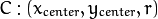出于上面提到的对运算效率的考虑, OpenCV实现的是一个比标准霍夫圆变换更为灵活的检测方法: 霍夫梯度法, 也叫2-1霍夫变换(21HT), 它的原理依据是圆心一定是在圆上的每个点的模向量上, 这些圆上点模向量的交点就是圆心, 霍夫梯度法的第一步就是找到这些圆心, 这样三维的累加平面就又转化为二维累加平面. 第二部根据所有候选中心的边缘非0像素对其的支持程度来确定半径. 21HT方法最早在Illingworth的论文The Adaptive Hough Transform中提出并详细描述, 也可参照Yuen在1990年发表的A Comparative Study of Hough Transform Methods for Circle Finding。 Bradski的《学习OpenCV》一书则对OpenCV中具体对算法的具体实现有详细描述并讨论了霍夫梯度法的局限性.

/// 转换成灰度
cvtColor( src, src_gray, CV_BGR2GRAY );

/// 减少噪音来避免错误的圆检测
GaussianBlur( src_gray, src_gray, Size(9, 9), 2, 2 );

vector<Vec3f> circles;

/// 使用霍夫变换去寻找圆
HoughCircles( src_gray, circles, CV_HOUGH_GRADIENT, 1, src_gray.rows/8, 200, 100, 0, 0 );

/// 将检测到的圆画出来
for( size_t i = 0; i < circles.size(); i++ )
{
Point center(cvRound(circles[i]), cvRound(circles[i]));
// circle center
circle( src, center, 3, Scalar(0,255,0), -1, 8, 0 );
// circle outline
circle( src, center, radius, Scalar(0,0,255), 3, 8, 0 );
}

/// 显示结果
namedWindow( "Hough Circle Transform Demo", CV_WINDOW_AUTOSIZE );
imshow( "Hough Circle Transform Demo", src );

1、remapping重映射

为了完成映射过程, 有必要获得一些插值为非整数像素坐标,因为源图像与目标图像的像素坐标不是一一对应的.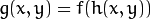这里 g（）是目标图像,f（） 是源图像,h(x,y) 是作用于(x,y) 的映射方法函数.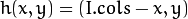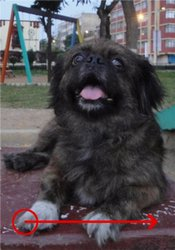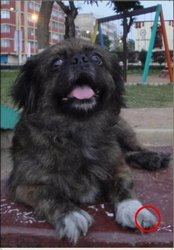/// Create dst, map_x and map_y with the same size as src:
dst.create( src.size(), src.type() );
map_x.create( src.size(), CV_32FC1 );
map_y.create( src.size(), CV_32FC1 );

 /// 下面是四种更新mapx和mapy的方法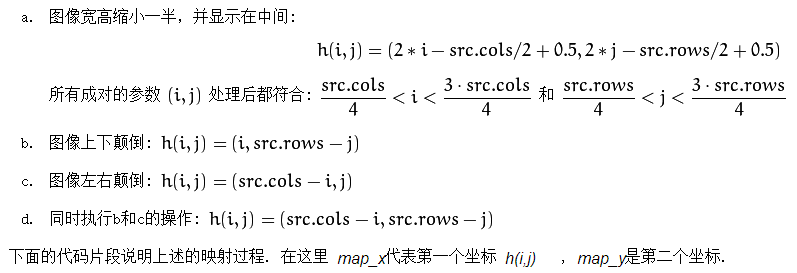ind = ind%4;

for( int j = 0; j < src.rows; j++ )
{ for( int i = 0; i < src.cols; i++ )
{
switch( ind )
{
case 0:
if( i > src.cols*0.25 && i < src.cols*0.75 && j > src.rows*0.25 && j < src.rows*0.75 )
{
map_x.at<float>(j,i) = 2*( i - src.cols*0.25 ) + 0.5 ;
map_y.at<float>(j,i) = 2*( j - src.rows*0.25 ) + 0.5 ;
}
else
{ map_x.at<float>(j,i) = 0 ;
map_y.at<float>(j,i) = 0 ;
}
break;
case 1:
map_x.at<float>(j,i) = i ;
map_y.at<float>(j,i) = src.rows - j ;
break;
case 2:
map_x.at<float>(j,i) = src.cols - i ;
map_y.at<float>(j,i) = j ;
break;
case 3:
map_x.at<float>(j,i) = src.cols - i ;
map_y.at<float>(j,i) = src.rows - j ;
break;
} // end of switch
}
}
ind++;

  remap( src, dst, map_x, map_y, CV_INTER_LINEAR, BORDER_CONSTANT, Scalar(0,0, 0) );


– INTER_NEAREST - 最近邻插值
– INTER_LINEAR - 双线性插值 (used by default)
– INTER_AREA -   使用像素区域相关的重采样。 它作为图像抽取的一个优先选择的方法，因为它可以得到moire’-free的结果.    不过当图像变焦（zoom，也是缩放的意思）的时候，这相似于INTER_NEAREST方法。
– INTER_CUBIC -  基于4×4的像素周边的双三次插值
– INTER_LANCZOS4 - 基于8×8的像素周边的Lanczos插值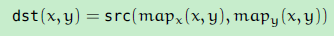2、仿射变换

我们能够用仿射变换来表示:旋转 (线性变换)；平移 (向量加)；缩放操作 (线性变换)。

事实上, 仿射变换代表的是两幅图之间的 关系 ， 我们通常使用 2x3的矩阵来表示仿射变换.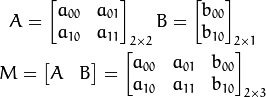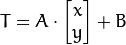或者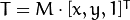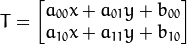仿射变换基本表示的就是两幅图片之间的 联系 . 关于这种联系的信息大致可从以下两种场景获得:

a、我们已知 XT 而且我们知道他们是有联系的. 接下来我们的工作就是求出矩阵 M

b、我们已知 M 和 X. 要想求得 T. 我们只要应用算式 T= M*X 即可. 对于这种联系的信息可以用矩阵 M 清晰的表达 (即给出明确的2×3矩阵) 或者也可以用两幅图片点之间几何关系来表达.

让我们形象地说明一下. 因为矩阵M 联系着两幅图片, 我们以其表示两图中各三点直接的联系为例. 见下图: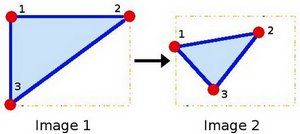点1, 2 和 3 (在图一中形成一个三角形) 与图二中三个点一一映射, 仍然形成三角形, 但形状已经大大改变. 如果我们能通过这样两组三点求出仿射变换 (你能选择自己喜欢的点), 接下来我们就能把仿射变换应用到图像中所有的点.

//定义一些需要用到的变量, 比如需要用来储存中间和目标图像的Mat和两个需要用来定义仿射变换的二维点数组

Point2f srcTri;//源图像的三个点
Point2f dstTri;//目标图像的三个点

Mat rot_mat( 2, 3, CV_32FC1 );//仿射旋转变换矩阵
Mat warp_mat( 2, 3, CV_32FC1 );//仿射变换矩阵
Mat src, warp_dst, warp_rotate_dst;//源图像、仿射变换的目标图像、仿射旋转的目标图
src = imread( argv, 1 );//加载源图像
warp_dst = Mat::zeros( src.rows, src.cols, src.type() );//以与源图像同样的类型和大小来对目标图像初始化
//仿射变换: 正如上文所说, 我们需要源图像和目标图像上分别一一映射的三个点来定义仿射变换:

srcTri = Point2f( 0,0 );
srcTri = Point2f( src.cols - 1, 0 );
srcTri = Point2f( 0, src.rows - 1 );

dstTri = Point2f( src.cols*0.0, src.rows*0.33 );
dstTri = Point2f( src.cols*0.85, src.rows*0.25 );
dstTri = Point2f( src.cols*0.15, src.rows*0.7 );
warp_mat = getAffineTransform( srcTri, dstTri );//通过这两组点, 我们能够使用OpenCV函数 getAffineTransform 来求出仿射变换，我们获得了用以描述仿射变换的 2×3矩阵 (在这里是 warp_mat)
warpAffine( src, warp_dst, warp_mat, warp_dst.size() );//将刚刚求得的仿射变换应用到源图像，这样我们就获得了变换后的图像! 我们将会把它显示出来
namedWindow( warp_window, CV_WINDOW_AUTOSIZE );
imshow( warp_window, warp_dst );//显示仿射变换的结果

Point center = Point( warp_dst.cols/2, warp_dst.rows/2 );
double angle = -50.0;//顺时针旋转50度
double scale = 0.6;//缩放因子
rot_mat = getRotationMatrix2D( center, angle, scale );//利用OpenCV函数 getRotationMatrix2D 来获得旋转矩阵, 这个函数返回一个2×3 矩阵 (这里是 rot_mat)
warpAffine( warp_dst, warp_rotate_dst, rot_mat, warp_dst.size() );//把旋转应用到仿射变换的输出
namedWindow( warp_rotate_window, CV_WINDOW_AUTOSIZE );
imshow( warp_rotate_window, warp_rotate_dst );//显示仿射旋转的结果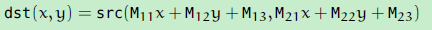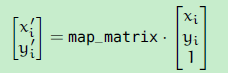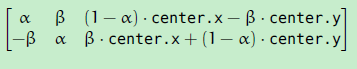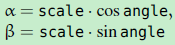posted @ 2015-01-06 22:19  仙守  阅读(940)  评论(0编辑  收藏  举报